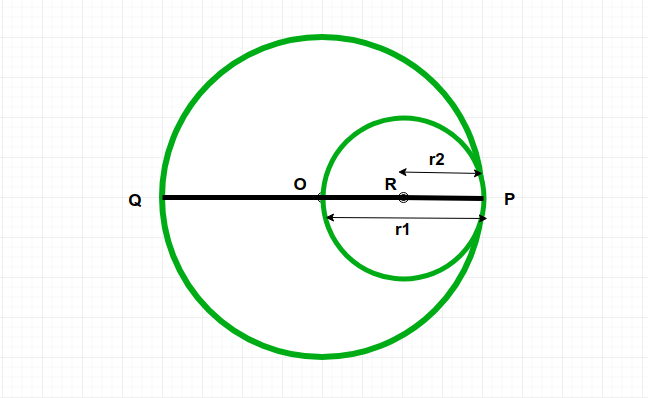Open in App
Not now

# Program to calculate area of inner circle which passes through center of outer circle and touches its circumference

• Difficulty Level : Hard
• Last Updated : 13 Sep, 2022

Given a circle C1 and it’s a radius r1. And one another circle C2 whose passes through center of circle C1 and touch the circumference of circle C1. The task is to find out the area of circle C2
Examples:

Input: r1 = 4
Output:Area of circle c2 = 12.56

Input: r1 = 7
Output:Area of circle c2 = 38.465Approach:
Radius r2 of circle C2 isSo we know that the area of circle is.
Below is the implementation of the above approach:

## C++

 // C++ implementation of the above approach #include  #include  using namespace std;   // Function calculate the area of the inner circle double innerCirclearea(double radius) {       // the radius cannot be negative     if (radius < 0)      {         return -1;     }       // area of the circle     double r = radius / 2;     double Area = (3.14 * pow(r, 2));       return Area; }   // Driver Code int main() {           double radius = 4;     cout << ("Area of circle c2 = ",                 innerCirclearea(radius));     return 0; }   // This code is contributed by jit_t.

## Java

 // Java implementation of the above approach   class GFG {       // Function calculate the area of the inner circle     static double innerCirclearea(double radius)     {           // the radius cannot be negative         if (radius < 0) {             return -1;         }           // area of the circle         double r = radius / 2;         double Area = (3.14 * Math.pow(r, 2));           return Area;     }       // Driver Code     public static void main(String arr[])     {         double radius = 4;         System.out.println("Area of circle c2 = "                            + innerCirclearea(radius));     } }

## Python3

 # Python3 implementation of the above approach    # Function calculate the area of the inner circle  def innerCirclearea(radius) :       # the radius cannot be negative      if (radius < 0) :         return -1;               # area of the circle     r = radius / 2;     Area = (3.14 * pow(r, 2));        return Area;        # Driver Code  if __name__ == "__main__" :           radius = 4;      print("Area of circle c2 =",             innerCirclearea(radius));    # This code is contributed by AnkitRai01

## C#

 // C# Implementation of the above approach using System;       class GFG  {       // Function calculate the area      // of the inner circle     static double innerCirclearea(double radius)     {           // the radius cannot be negative         if (radius < 0)          {             return -1;         }           // area of the circle         double r = radius / 2;         double Area = (3.14 * Math.Pow(r, 2));           return Area;     }       // Driver Code     public static void Main(String []arr)     {         double radius = 4;         Console.WriteLine("Area of circle c2 = " +                            innerCirclearea(radius));     } }    // This code is contributed by PrinciRaj1992

## Javascript

 

Output:

Area of circle c2 = 12.56

Time Complexity : O(1) ,as it is doing constant operations

Auxiliary Space : O(1) ,as we are not using any extra space.

My Personal Notes arrow_drop_up
Related Articles